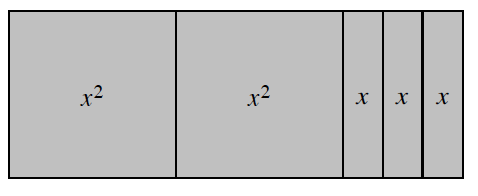### Home > CC2MN > Chapter 4 > Lesson 4.3.1 > Problem4-75

4-75.

Sketch the shape made with algebra tiles at right on your paper. Then answer parts (a) and (b) below.

1. Find the area and perimeter of the shape.

To find the total area, add together the area of the algebra tiles.
$x^2+x^2+x+x+x$

To find the perimeter, label all the sides with their lengths, and then add them together.
$x+x+x+x+x+x+1+1+1+1+1+1$

Area: $2x^2+3x$
Perimeter: $6x+6$

2. If the algebra tiles were rearranged into a different shape, how would the area change?

Think about how you determined the area. Would rearranging the tiles change their individual areas or the total area of the shape? Rearrange the tiles into a new shape and see how it changes the area.Use the tiles in the eTool below to create the shape.
Click the link at right for the full version of the eTool: 4-75 HW eTool (CPM)